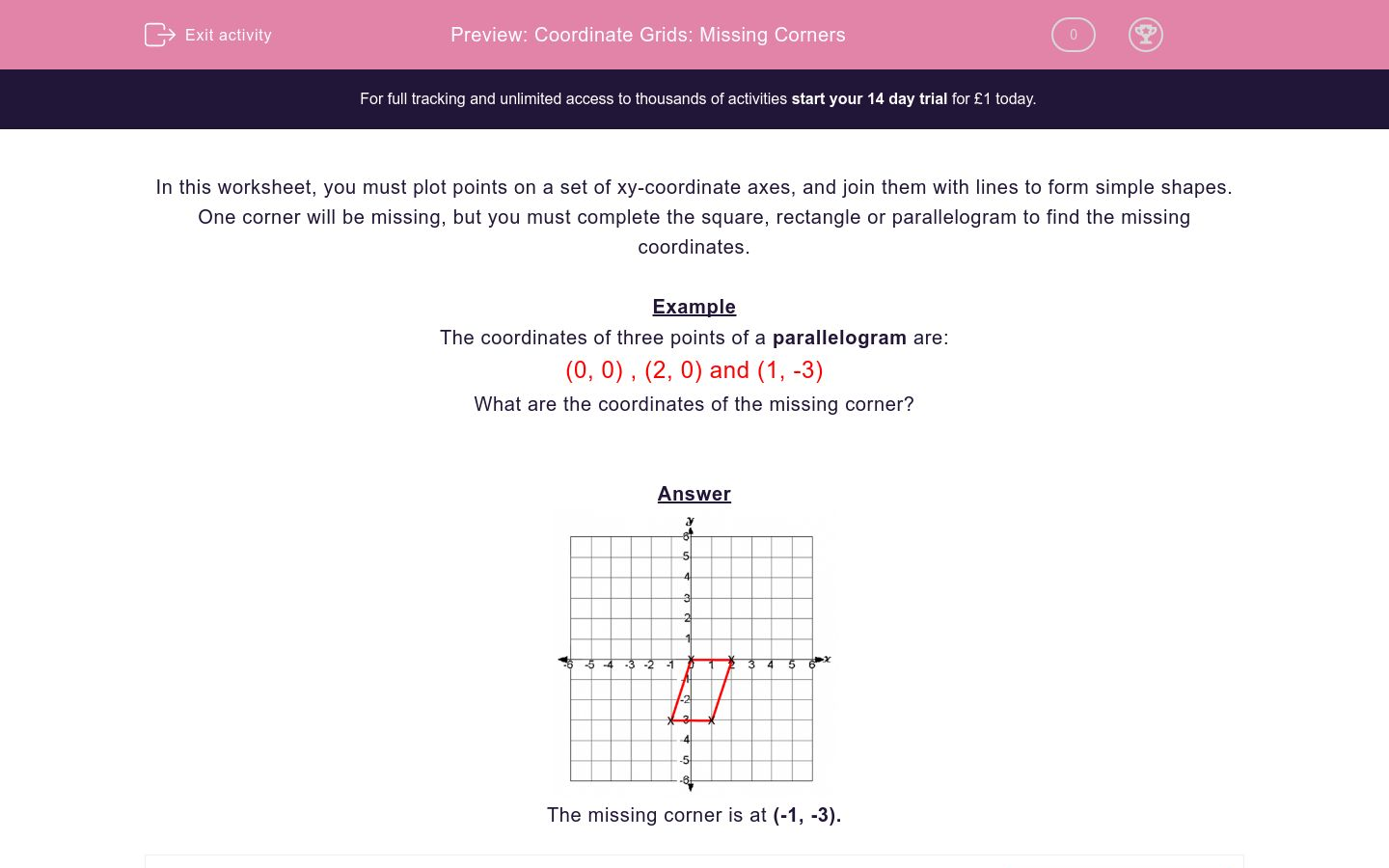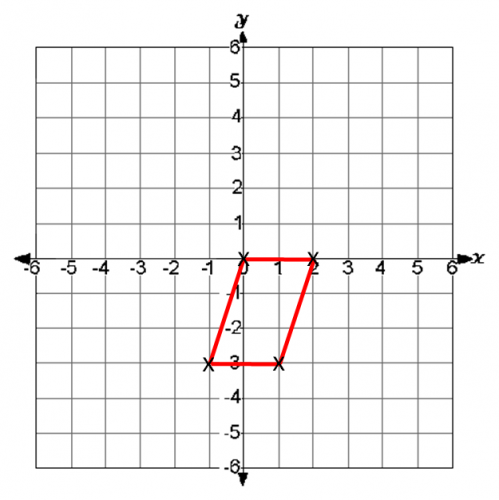# Coordinate Grids: Missing Corners

In this worksheet, students must state the coordinates of the missing corner of the given quadrilaterals.Key stage:  KS 2

Curriculum topic:   Maths and Numerical Reasoning

Curriculum subtopic:   2D Shapes: Triangles, Quadrilaterals and Polygons

Difficulty level:#### Worksheet Overview

In this worksheet, you must plot points on a set of xy-coordinate axes, and join them with lines to form simple shapes.

One corner will be missing, but you must complete the square, rectangle or parallelogram to find the missing coordinates.

Example

The coordinates of three points of a parallelogram are:

(0, 0) , (2, 0) and (1, -3)

What are the coordinates of the missing corner?